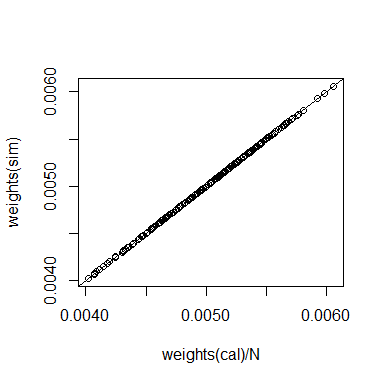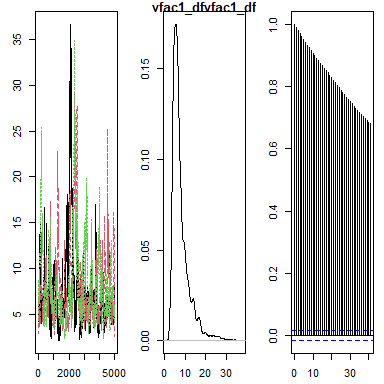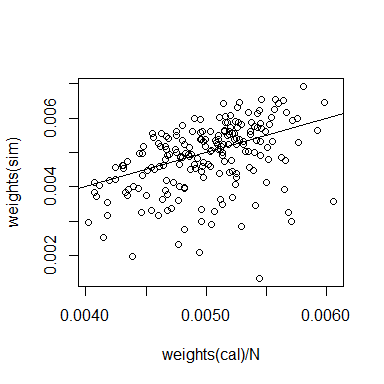# Linear regression, prediction, and survey weighting

We use the api dataset from package survey to illustrate estimation of a population mean from a sample using a linear regression model. First let’s estimate the population mean of the academic performance indicator 2000 from a simple random sample, apisrs. Using package survey’s GREG estimator (Särndal, Swensson, and Wretman 1992), we find

library(survey)
data(api)
# define the regression model
model <- api00 ~ ell + meals + stype + hsg + col.grad + grad.sch
# compute corresponding population totals
XpopT <- colSums(model.matrix(model, apipop))
N <- XpopT[["(Intercept)"]]  # population size
# create the survey design object
des <- svydesign(ids=~1, data=apisrs, weights=~pw, fpc=~fpc)
# compute the calibration or GREG estimator
cal <- calibrate(des, formula=model, population=XpopT)
svymean(~ api00, des)  # equally weighted estimate
##         mean     SE
## api00 656.58 9.2497
svymean(~ api00, cal)  # GREG estimate
##         mean     SE
## api00 663.86 4.1942

The true population mean in this case can be obtained from the apipop dataset:

mean(apipop$api00) ##  664.7126 Note that the GREG estimate is more accurate than the simple equally weighted estimate, which is also reflected by the smaller estimated standard error of the former. We can run the same linear model using package mcmcsae. In the next code chunk, function create_sampler sets up a sampler function that is used as input to function MCMCsim, which runs a simulation to obtain draws from the posterior distribution of the model parameters. By default three chains with independently generated starting values are run over 1250 iterations with the first 250 discarded as burnin. As the starting values for the MCMC simulation are randomly generated, we set a random seed for reproducibility. The results of the simulation are subsequently summarized, and the DIC model criterion is computed. The simulation summary shows several statistics for the model parameters, including the posterior mean, standard error, quantiles, as well as the R-hat convergence diagnostic. library(mcmcsae) set.seed(1) sampler <- create_sampler(model, data=apisrs) sim <- MCMCsim(sampler, verbose=FALSE) (summ <- summary(sim)) ## llh_ : ## Mean SD t-value MCSE q0.05 q0.5 q0.95 n_eff R_hat ## llh_ -1104 2.12 -520 0.0418 -1108 -1104 -1101 2581 1 ## ## sigma_ : ## Mean SD t-value MCSE q0.05 q0.5 q0.95 n_eff R_hat ## sigma_ 60.5 3.11 19.4 0.0631 55.5 60.4 66 2433 1 ## ## reg1 : ## Mean SD t-value MCSE q0.05 q0.5 q0.95 n_eff R_hat ## (Intercept) 778.32 24.600 31.64 0.44912 737.311 778.33 818.5935 3000 1.000 ## ell -1.72 0.298 -5.79 0.00544 -2.210 -1.72 -1.2382 3000 1.000 ## meals -1.75 0.275 -6.36 0.00502 -2.209 -1.75 -1.3115 3000 1.000 ## stypeH -108.81 14.023 -7.76 0.25603 -131.403 -108.60 -86.1115 3000 1.002 ## stypeM -59.05 12.117 -4.87 0.22122 -78.968 -59.08 -39.2607 3000 0.999 ## hsg -0.70 0.415 -1.69 0.00758 -1.408 -0.70 -0.0101 3000 1.000 ## col.grad 1.22 0.487 2.51 0.00889 0.421 1.22 2.0163 3000 1.000 ## grad.sch 2.20 0.501 4.39 0.00937 1.402 2.19 3.0274 2859 1.000 compute_DIC(sim) ## DIC p_DIC ## 2217.368326 8.910283 The output of function MCMCsim is an object of class mcdraws. The package provides methods for the generic functions summary, plot, predict, residuals and fitted for this class. ## Prediction To compute a model-based estimate of the population mean, we need to predict the values of the target variable for the unobserved units. Let $$U$$ denote the set of population units, $$s \subset U$$ the set of sample (observed) units, and let $$y_i$$ denote the value of the variable of interest taken by the $$i$$th unit. The population mean of the variable of interest is $\bar{Y} = \frac{1}{N}\sum_{i=1}^N y_i = \frac{1}{N}\left(\sum_{i\in s} y_i + \sum_{i\in U\setminus s} y_i \right)\,.$ Posterior draws for $$\bar{Y}$$ can be obtained by generating draws for the non-sampled population units, summing them and adding the sample sum. This is done in the next code chunk, where method predict is used to generate draws from the posterior predictive distribution for the new, unobserved, units. m <- match(apisrs$cds, apipop$cds) # population units in the sample # use only a sample of 250 draws from each chain predictions <- predict(sim, newdata=apipop[-m, ], iters=sample(1:1000, 250), show.progress=FALSE) str(predictions) ## List of 3 ##$ : num [1:250, 1:5994] 699 623 586 599 608 ...
##  $: num [1:250, 1:5994] 704 672 619 746 692 ... ##$ : num [1:250, 1:5994] 587 701 743 673 582 ...
##  - attr(*, "class")= chr "dc"
samplesum <- sum(apisrs$api00) summary(transform_dc(predictions, fun = function(x) (samplesum + sum(x))/N)) ## Mean SD t-value MCSE q0.05 q0.5 q0.95 n_eff R_hat ## [1,] 664 4.12 161 0.151 657 664 671 750 1 The result for the population mean can also be obtained directly (which is more efficient memory wise) by supplying the appropriate aggregation function to the prediction method: summary(predict(sim, newdata=apipop[-m, ], fun=function(x, p) (samplesum + sum(x))/N, show.progress=FALSE) ) ## Mean SD t-value MCSE q0.05 q0.5 q0.95 n_eff R_hat ## [1,] 664 4.21 158 0.083 657 664 671 2565 0.999 For any linear model one can obtain the same result more efficiently by precomputing covariate population totals. Posterior draws for $$\bar{Y}$$ are then computed as $\bar{Y}_r = \frac{1}{N} \left( n\bar{y} + \beta_r'(X - n\bar{x}) + e_r\right)\,,$ where $$e_r \sim {\cal N}(0, (N-n)\sigma_r^2)$$, representing the sum of $$N-n$$ independent normal draws. The code to do this is n <- nrow(apisrs) XsamT <- colSums(model.matrix(model, apisrs)) XpopR <- matrix(XpopT - XsamT, nrow=1) predictions <- predict(sim, X=list(reg1=XpopR), var=N-n, fun=function(x, p) (samplesum + x)/N, show.progress=FALSE) summary(predictions) ## Mean SD t-value MCSE q0.05 q0.5 q0.95 n_eff R_hat ## [1,] 664 4.2 158 0.0797 657 664 671 2782 0.999 ## Weights To compute weights corresponding to the population total: sampler <- create_sampler(model, data=apisrs, linpred=list(reg1=matrix(XpopT/N, nrow=1)), compute.weights=TRUE) sim <- MCMCsim(sampler, verbose=FALSE) plot(weights(cal)/N, weights(sim)); abline(0, 1)sum(weights(sim) * apisrs$api00)
##  663.8594
print(summary(sim, "linpred_"), digits=6)
## linpred_ :
##             Mean      SD t-value      MCSE   q0.05    q0.5   q0.95 n_eff    R_hat
## linpred_ 663.792 4.35517 152.415 0.0795141 656.689 663.682 671.264  3000 0.999854

Note the small difference between the weighted sample sum of the target variable and the posterior mean of the linear predictor. This is due to Monte Carlo error; the weighted sum is exact for the simple linear regression case.

## Outliers

One possible way to deal with outliers is to use a Student-t sampling distribution, which has fatter tails than the normal distribution. In the next example, the formula.V argument is used to add local variance parameters with inverse chi-squared distributions. The marginal sampling distribution then becomes Student-t. Here the degrees of freedom parameter is modeled, i.e. assigned a prior distribution and inferred from the data.

sampler <- create_sampler(model, formula.V=~vfac(prior=pr_invchisq(df="modeled")),
linpred=list(reg1=matrix(XpopR/N, nrow=1)),
data=apisrs, compute.weights=TRUE)
sim <- MCMCsim(sampler, n.iter=5000, burnin=1000, verbose=FALSE)
(summ <- summary(sim))
## llh_ :
##       Mean   SD t-value  MCSE q0.05  q0.5 q0.95 n_eff R_hat
## llh_ -1081 8.15    -133 0.605 -1094 -1081 -1067   181  1.01
##
## sigma_ :
##        Mean   SD t-value  MCSE q0.05 q0.5 q0.95 n_eff R_hat
## sigma_ 49.5 4.39    11.3 0.304  42.5 49.4  56.9   209  1.01
##
## linpred_ :
##          Mean  SD t-value   MCSE q0.05 q0.5 q0.95 n_eff R_hat
## linpred_  643 3.9     165 0.0402   636  643   649  9435     1
##
## reg1 :
##                 Mean     SD t-value    MCSE    q0.05     q0.5    q0.95 n_eff R_hat
## (Intercept)  793.917 26.129   30.38 0.37692  750.021  794.556 836.0596  4806     1
## ell           -1.485  0.362   -4.10 0.00751   -2.081   -1.491  -0.8870  2329     1
## meals         -2.083  0.358   -5.82 0.00889   -2.681   -2.077  -1.5006  1621     1
## stypeH      -105.409 12.826   -8.22 0.12953 -126.374 -105.356 -83.9533  9806     1
## stypeM       -56.837 10.943   -5.19 0.10842  -74.898  -56.824 -38.6756 10187     1
## hsg           -0.677  0.454   -1.49 0.00562   -1.419   -0.676   0.0663  6524     1
## col.grad       0.967  0.478    2.02 0.00558    0.199    0.963   1.7661  7342     1
## grad.sch       2.114  0.467    4.53 0.00508    1.346    2.110   2.8863  8440     1
##
## vfac1_df :
##          Mean   SD t-value MCSE q0.05 q0.5 q0.95 n_eff R_hat
## vfac1_df 7.74 4.43    1.75 0.49  3.62 6.36  16.6  81.6  1.03
plot(sim, "vfac1_df")acceptance_rates(sim)
## []
## [][]
##  0.2642
##
## [][]
##  0.2692
##
## [][]
##  0.2648
compute_DIC(sim)
##       DIC     p_DIC
## 2194.8432   33.7317
predictions <- predict(sim, newdata=apipop[-m, ], show.progress=FALSE,
fun=function(x, p) (samplesum + sum(x))/N)
summary(predictions)
##      Mean   SD t-value   MCSE q0.05 q0.5 q0.95 n_eff R_hat
## [1,]  664 3.98     167 0.0405   657  664   670  9659     1
plot(weights(cal)/N, weights(sim)); abline(0, 1)summary(get_means(sim, "Q_")[["Q_"]])
##    Min. 1st Qu.  Median    Mean 3rd Qu.    Max.
##  0.2103  0.9318  1.0747  1.0003  1.1272  1.1665